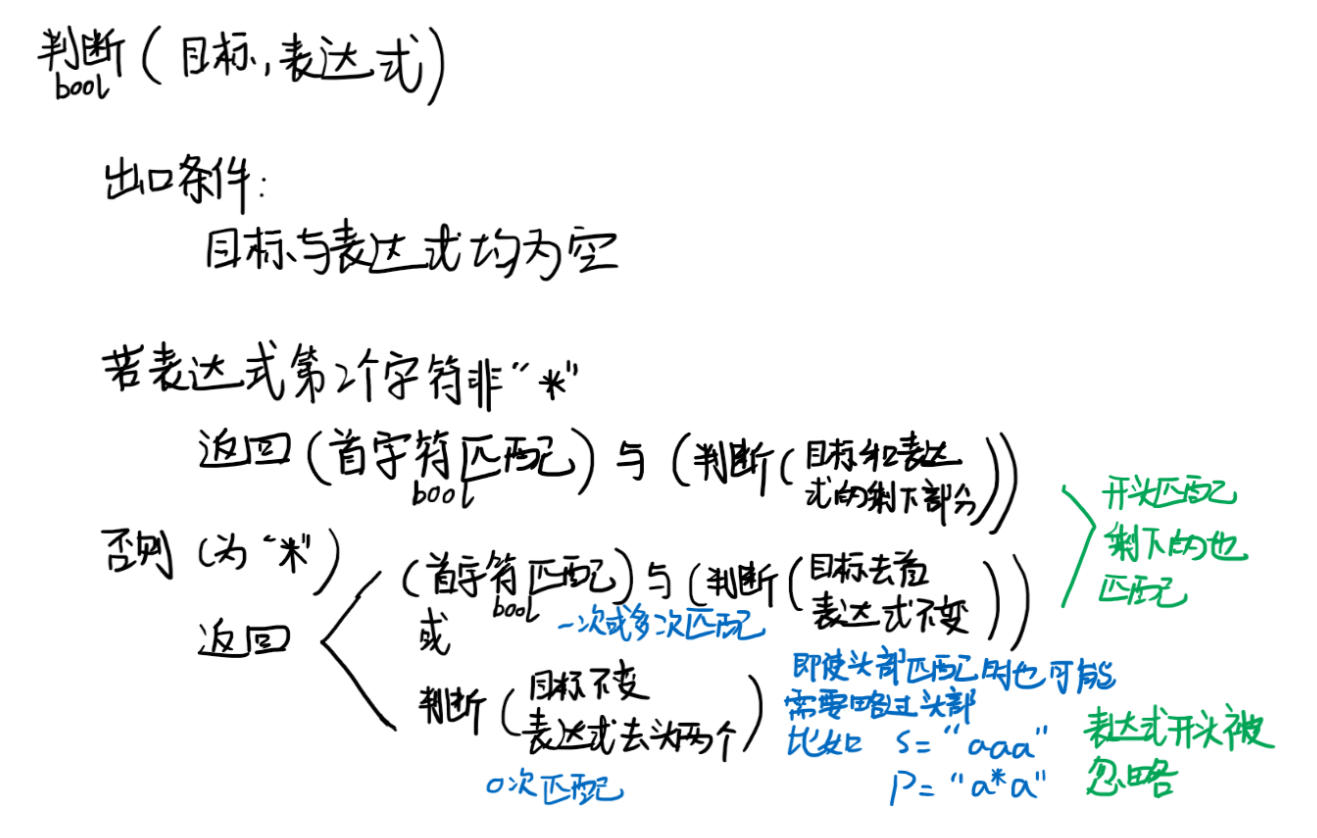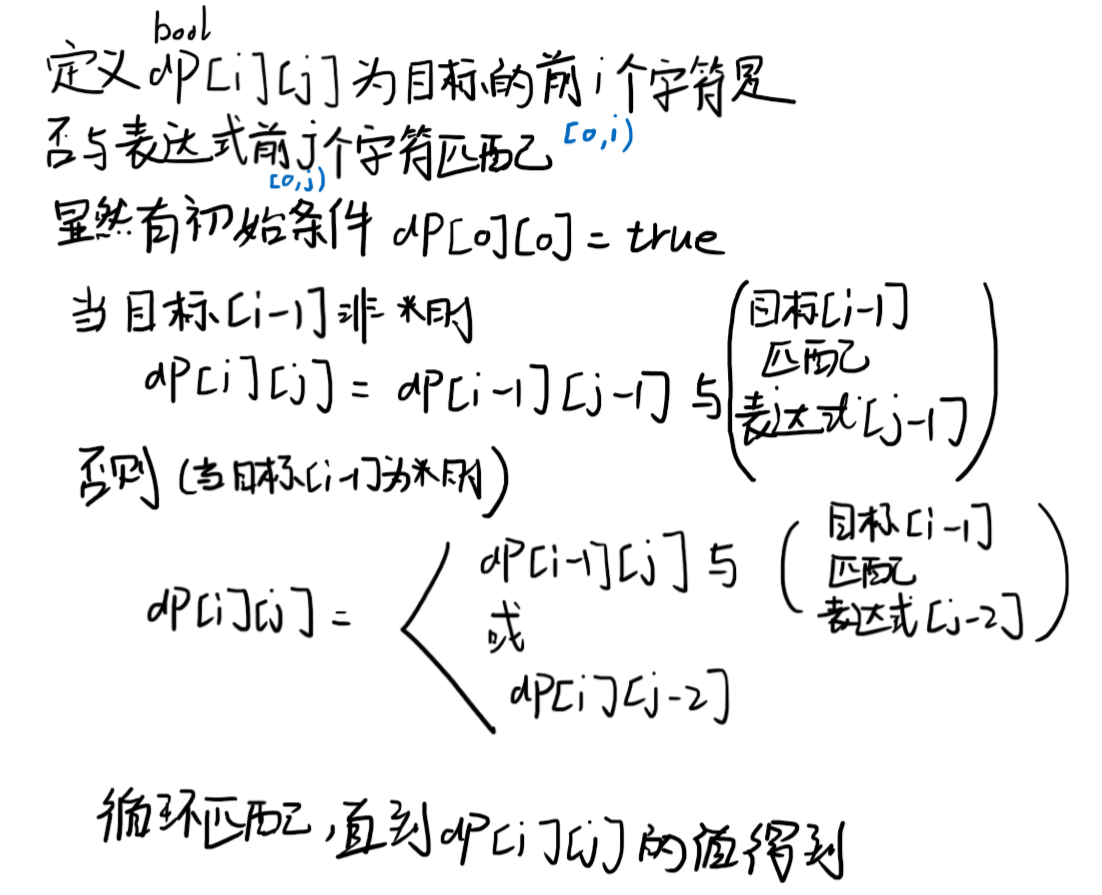# 背景

``````给你一个字符串 s 和一个字符规律 p，请你来实现一个支持 '.' 和 '*' 的正则表达式匹配。

'.' 匹配任意单个字符
'*' 匹配零个或多个前面的那一个元素

``````示例 1：

# 好思路

## 递归``````bool isMatch(const char* s, const char* p) {
if(*p == 0) return *s == 0;

bool first_match = *s && (*s == *p || *p == '.');

if(*(p+1) == '*'){
return isMatch(s, p+2) || (first_match && isMatch(++s, p));
}
else{
return first_match && isMatch(++s, ++p);
}
}``````

``````class Solution {
private:
const char *s, *p;
int sz_s, sz_p;
int *result;
public:
bool isMatch(string s, string p) {
this->s = s.c_str();
this->p = p.c_str();
sz_s = s.length();
sz_p = p.length();
result = (int *)malloc((sz_s+1) * (sz_p+1) * sizeof(int));
memset(result, 0, sz_s * sz_p * sizeof(int));
return isMatch(this->s, this->p);
}

bool isMatch(const char* s, const char* p) {
int s_index = s - this->s;
int p_index = p - this->p;

if(*p == 0) return *s == 0;

if (result[s_index * sz_p +p_index]==1)
return true;
else if(result[s_index * sz_p +p_index]==-1)
return false;

auto first_match = *s && (*s == *p || *p == '.');

if(*(p+1) == '*'){
if(isMatch(s, p+2) || (first_match && isMatch(++s, p))){
result[s_index * sz_p +p_index] = 1;
return true;
}
result[s_index * sz_p +p_index] = -1;
return false;
}
else{
if(first_match && isMatch(++s, ++p)){
result[s_index * sz_p +p_index] = 1;
return true;
}
result[s_index * sz_p +p_index] = -1;
return false;
}
}
};``````

## 动态规划``````class Solution {
public:
bool isMatch(string s, string p) {
int m = s.size(), n = p.size();
vector<vector<bool>> dp(m + 1, vector<bool>(n + 1, false));
dp = true;
for (int i = 0; i <= m; i++) {
for (int j = 1; j <= n; j++) {
if (p[j - 1] == '*') {
dp[i][j] = dp[i][j - 2] || (i && dp[i - 1][j] && (s[i - 1] == p[j - 2] || p[j - 2] == '.'));
} else {
dp[i][j] = i && dp[i - 1][j - 1] && (s[i - 1] == p[j - 1] || p[j - 1] == '.');
}
}
}
return dp[m][n];
}
};``````

i在循环的外层确保了`dp[i - 1][j]`的值已经提前计算好了